GRE Subject Test: Chemistry : Orbitals and Bonding

Example Questions

Example Question #1 : Quantum Numbers

Which of the following sets of quantum numbers (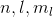) could correspond to a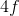orbital?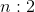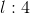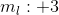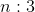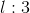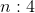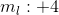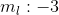Explanation:

Since we're looking at theorbital, we know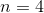. The range of possible values foris 0 to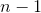. Possible values for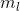range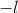to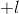. Therefore, among the answer choices,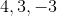is the only possible combination of quantum numbers corresponding to anorbital.

Example Question #2 : Quantum Numbers

Which of the following sets of quantum numbers could correspond to a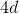orbital?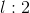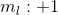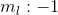Explanation:

Since we're looking at theorbital, we know. The range of possible values foris 0 to. Possible values forrangeto. Therefore, among the answer choices,is the only possible combination of quantum numbers corresponding to aorbital.

All GRE Subject Test: Chemistry Resources